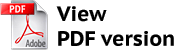https://vestnik.volbi.ru/
﻿

# COMPARATIVE ANALYSIS OF METHODS OF CALCULATING PRODUCTION PRIME COST

Back to articles of current journal
 PDF:Author(s): Kiseleva M. M., Yatsko V. A., Number of journal: 4(41) Date: November 2017 Annotation: On the basis of comparative analysis of different methods of calculating the prime cost, the article shows that all known methods of calculation of the full prime cost is inaccurate. Under certain conditions this situation can lead to inefficient management decisions related to pricing, the management of range of products, business restructurisation. For the purpose of taking managerial decisions when analyzing the prime cost of production, the article offers to use an approach based on the apparatus of the theory of fuzzy sets. As a result of using this approach, the prime cost is represented as a fuzzy number that integratively represents the possible values of the prime cost. Keywords: expenses, prime cost, calculating, profitability, fixed costs, variable costs, cost allocation, cost allocation base, full prime cost, partial prime cost, fuzzy set. For citation: Kiseleva M. M., Yatsko V. A. Comparative analysis of methods of calculating production prime cost // Business. Education. Law. 2017. No. 4 (41). P. 141–144.